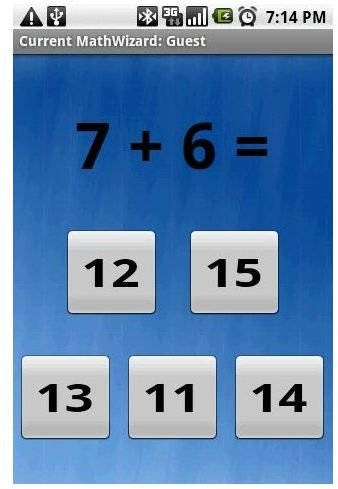# Best Math Software for Android

Page content

## Android Math Apps to Improve Your Math Skills

Arithmetic sequences, algebraic equations, statistics, trigonometry or basic addition and subtraction - every math problem may seem quite challenging to mathematics students at first. What they need is a handy reference tool to help them solve complex equations. There are many helpful Android apps that provide math hints, tips and tricks to students who wish to excel in mathematics. The best math software for Android also presents tricky quizzes, and fun arithmetic mini-games that help sharpen the brain and test mental math. Here are some of the best math applications for the Android platform that test the math skills of both elementary and high school students:

## Math Reference

A great reference tool for both elementary and high school students, this Android math app provides easy access to a variety of math formulas and complex equations, be it basic arithmetic, statistics, algebra, geometry, conversions, probability or series and sequences.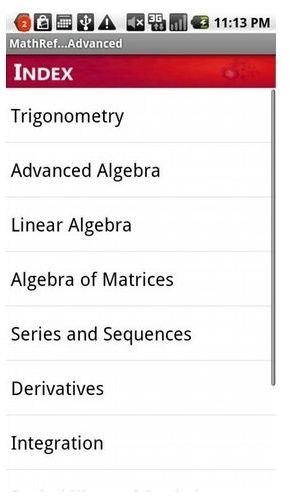In Math Reference, you will notice two options: Basic and Advanced. The Basic option covers elementary mathematics whereas the advanced option covers trigonometry, integration and other advanced math. Select the option and get simple guides and hints on solving complex math problems. The interface is simple and easy-to-use, but there should have been a search bar to search for the required formula or topic. Overall, a very useful app that truly provides a quick reference to all your math problems. The application is available for \$0.99.

Math Reference QR Code

## Kids Numbers and Math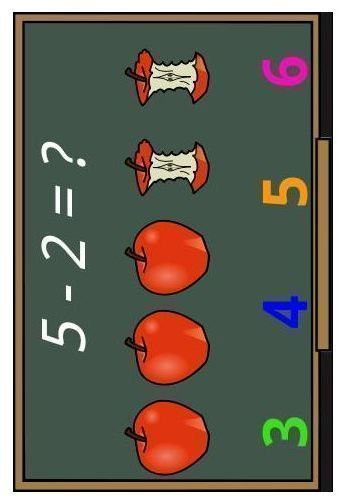This math software for Android can really help preschoolers get familiar with numbers and develop their pre-math skills. Kids can easily learn addition, subtraction and choose the minimum and maximum numbers as well as spell numbers prompted by a voice. With nice hand-drawn cartoony visuals and voice prompts, the application improves your child’s counting skills as well as basic addition and subtraction.

## Math Workout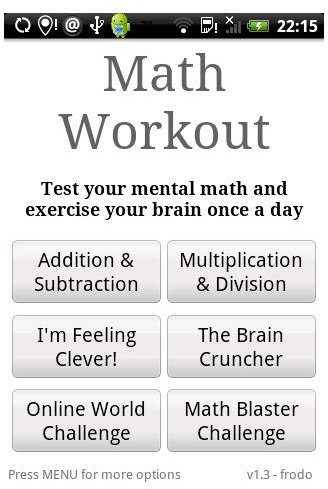Designed to improve your child’s arithmetic skills, Math Workout presents several mini-games such as Math Blaster, World Challenge etc. that truly help sharpen your child’s mental math. Besides the basic math workout games, the fun mini-games truly enhance the mental arithmetic of kids, teens and even grown-ups.

If you want a fun way to learn arithmetic, then try this application. You can first use the lite version and if you like it then buy the Pro version. You’ll find it has many additional cool features, a progress chart that keeps track of the math exercises and games completed successfully along with additional workouts.

## Ultimath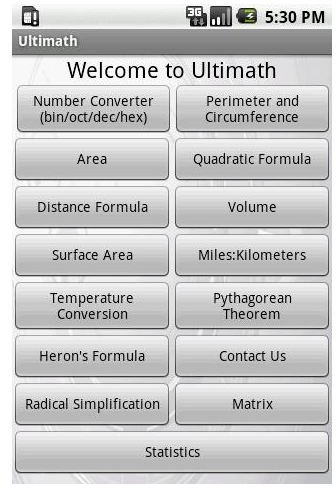Tricky math problems always haunt students, don’t they? But now, there is excellent math software for Android that allows students to find solutions to complex math problems in no time. Whether it is a difficult Heron’s formula or plain number and temperature converter, students will find everything in one place. There are plenty of surface area, volume, area and perimeter quick solvers (things that I hated during my school days). Students can solve Pythagorean equations using its nifty graphical solver.

The application is available free and there may be some additional changes to bring up some new features in the days to come. Overall, a great app for those who really find it hard to solve complex math equations.